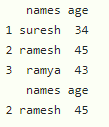# How to Use “NOT IN” Operator in R?

• Last Updated : 19 Dec, 2021

In this article, we will discuss NOT IN Operator in R Programming Language.

NOT IN Operator is used to check whether the element in present or not. The symbol used for IN operator is “%in%”. For NOT IN operator we have to add ” ! ” operator before that , so the symbol for NOT IN operator is “! %in%”.

## Method 1: Use “NOT IN” with Vectors

Here we are going to use this operator in a vector to select the elements that are not in particular elements.

Syntax:

`vector[!(vector %in% c(values))]`

where,

• vector is an input vector
• values are the values to be checked

Example:

## R

 `# vector``vector1 = ``c``(23, 34, 56, 23, 16, 78, ``            ``56, 4, 5, 6, 7, 8)`` ` `# display``print``(vector1)`` ` `# get the elements from a vector not in the values``print``(vector1[!(vector1 % ``in` `% ``c``(23, 34, 56, 5, 6, 7))])`

Output:

``` 23 34 56 23 16 78 56  4  5  6  7  8
 16 78  4  8```

## Method 2: Use “NOT IN” with DataFrames

Here we are going to use this filter in dataframe. We can select the values based on the column using this operator using subset function.

Syntax:

`subset(dataframe, !(column_name %in% c(values)))`

Where,

• dataframe is the input dataframe
• values are values to be checked for selection

Example:

## R

 `# create a dataframe``data = ``data.frame``(names=``c``(``"suresh"``, ``"ramesh"``, ``"ramya"``),``                  ``age=``c``(34, 45, 43))`` ` `# display``print``(data)`` ` `# get the elements from a dataframe names ``# column not in the values``print``(``subset``(data, !(names % ``in` `% ``c``(``'suresh'``, ``'ramya'``))))`

Output:My Personal Notes arrow_drop_up